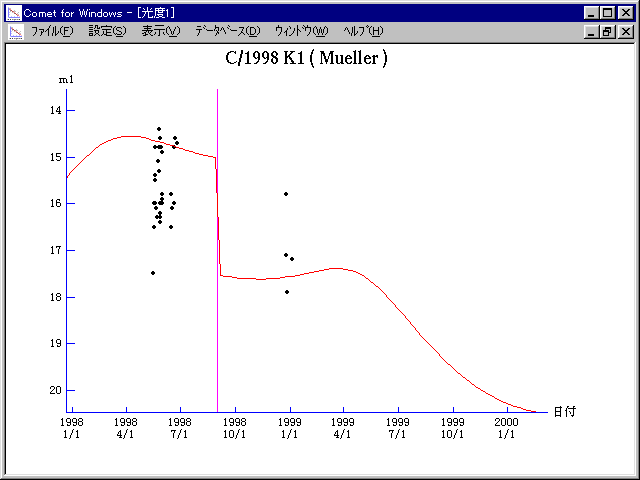# \$B%_%e%i!

C/1998 K1 ( Mueller )###\$B%W%m%U%#!<%k(B

 \$BH/8+F|(B 1998\$BG/(B5\$B7n(B16\$BF|(B \$BH/8+8wEY(B 17.5\$BEy(B \$BH/8+ Jean Mueller

###\$B###\$B50F;MWAG(B

```   The following improved orbital elements by Kenji Muraoka, are
from 160 observations 1998 Apr. 22  to  Dec. 27, perturbations
by 9 Planets, Moon and 5 minor planets were taken into account.
The mean residual is +/- 0.56 arc seconds.

Epoch  =  1998 Aug. 15.0  TT       JDT = 2451040.5
T  =  1998 Sept. 1.01935       +/- 0.00334 (m.e.) TT
Peri. =  165.24641                +/- 0.00085
Node  =   18.25555                +/- 0.00066   (2000.0)
Incl. =   35.63678                +/- 0.00026
q  =    3.4166407              +/- 0.0000219 AU
e  =    0.9451411              +/- 0.0000595
1/a  =   +0.0160564              +/- 0.0000174 1/AU
( P  =    492 years )
```

###\$B@1?^(B###\$B8wEYJQ2=(B

```        m1 = 6.5 + 5 log\$B&\$(B + 10.0 log r  [ ,0]  (              \$B!A(B1998\$BG/(B 8\$B7n(B31\$BF|(B)
m1 = 9.0 + 5 log\$B&\$(B + 10.0 log r  [0, ]  (1998\$BG/(B 8\$B7n(B31\$BF|!A(B              )
```##### \$B50F;MWAG\$OB<2,7r<#;a\$N7W;;\$K\$h\$k\$b\$N\$G\$9!#(B \$B@1?^\$O(B StellaNavigator Ver.2.0 for Windows (\$B%"%9%H%m%"!<%D(B \$BJTCx(B / \$B%"%9%-!<=PHG6I4)(B) \$B\$G:n@.\$7\$?\$b\$N\$G\$9!#(B \$B8wEY%0%i%U\$O(BComet for Windows\$B\$G:n@.\$7\$?\$b\$N\$G\$9!#(B